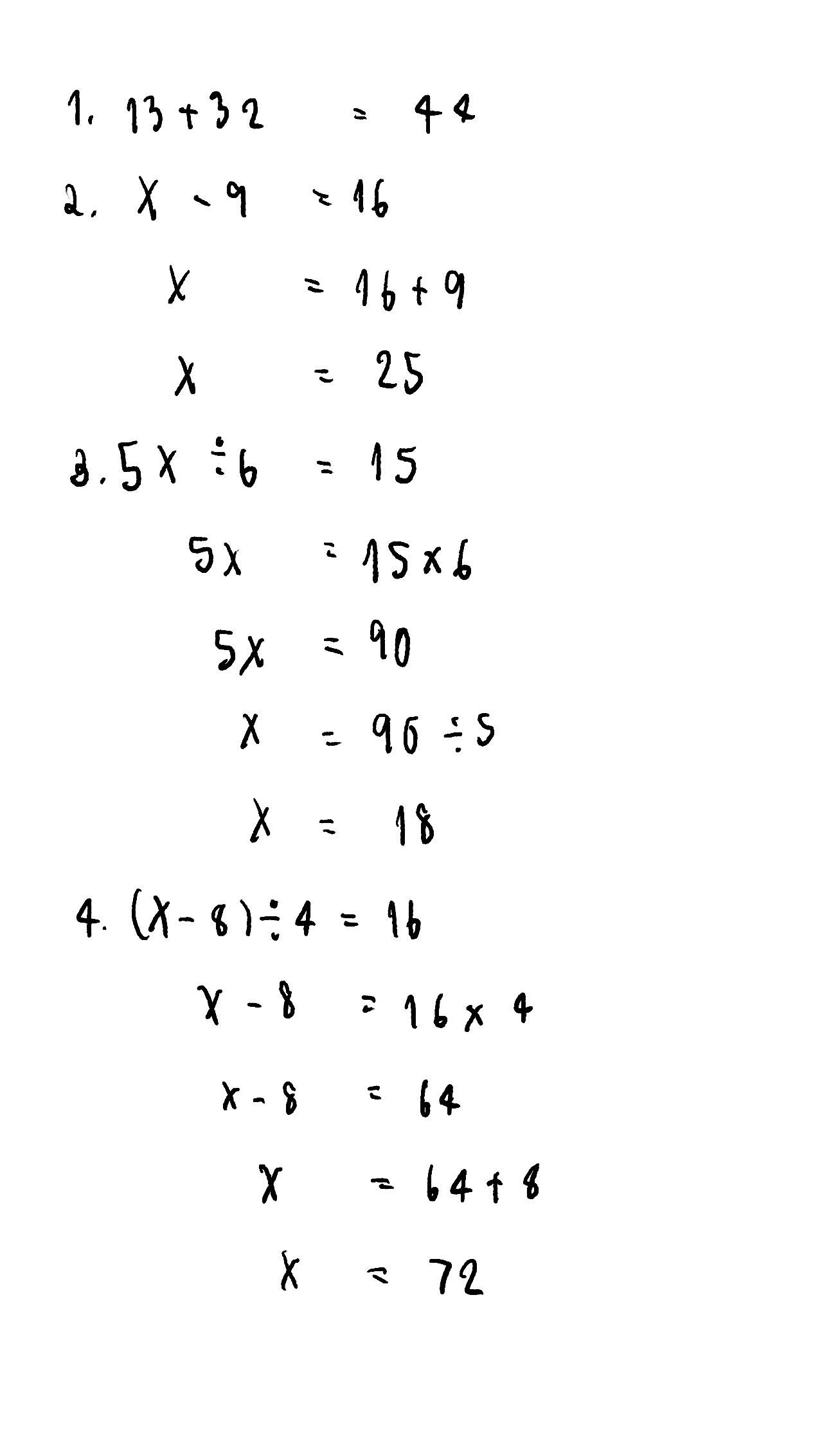เครื่องหมาย
โจทย์ปัญหา$11.$ Solve the following problems. $\left(a\right)$ The sum of a number and $13$ is $32.$ Find the number. (b) $b\right)$ The difference between x and $9$ $is$ $16,$ and x is greater than $9$ Find the value of $x$ (C) A number is multiplied by 5 and then divided by $6$ The result $1s$ $15$ Find the ทนmber. $\left(O\right)$ $8$ is subtracted from a number, then divided by $4$ The result $1S$ $6.$ Find the nนmber,
มัธยมต้น
คณิตศาสตร์
วิธีการแก้ปัญหาคุณครู Qanda - ฝายนักเรียน
ขอบคุณค่ะ?????คุณครู Qanda - ฝาย
ไม่เป็นไรค่ะ
ยังไม่เข้าใจใช่ไหม?
ถามคำถามกับครู Qanda